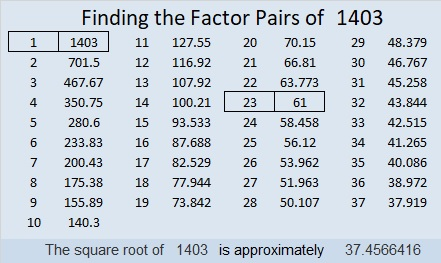# 1403 Multiplication Table Challenge

Just because you’re not in elementary school anymore doesn’t mean that the multiplication table can’t be a challenge. This one certainly is. Can you write the numbers 1 to 10 in the four factor areas so that this multiplication table works with the given clues? Don’t get discouraged; it will probably take you at least 15 minutes just to put those factors in the right places.Print the puzzles or type the solution in this excel file: 12 Factors 1389-1403

Now I’ll share some information about the puzzle number, 1403:

• 1403 is a composite number.
• Prime factorization: 1403 = 23 × 61.
• 1403 has no exponents greater than 1 in its prime factorization, so √1403 cannot be simplified.
• The exponents in the prime factorization are 1, and 1. Adding one to each exponent and multiplying we get (1 + 1)(1 + 1) = 2 × 2 = 4. Therefore 1403 has exactly 4 factors.
• The factors of 1403 are outlined with their factor pair partners in the graphic below.1403 is the hypotenuse of a Pythagorean triple:
253-1380-1403 which is 23 times (11-60-61)

This site uses Akismet to reduce spam. Learn how your comment data is processed.